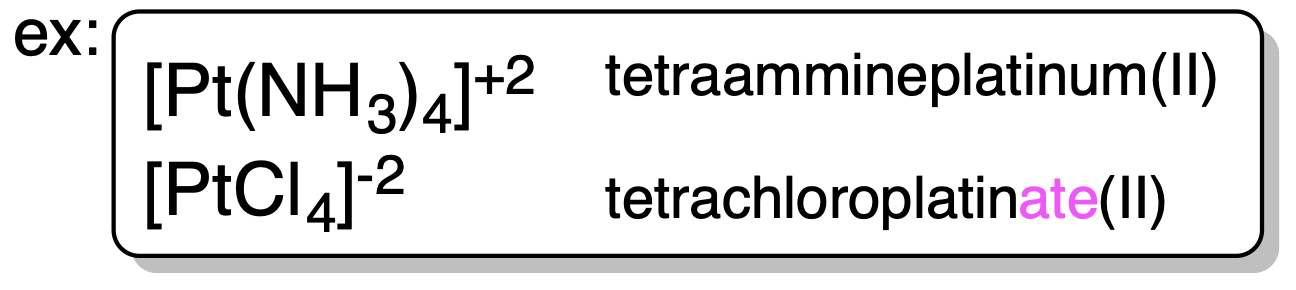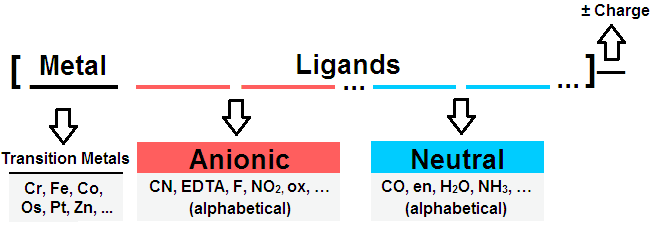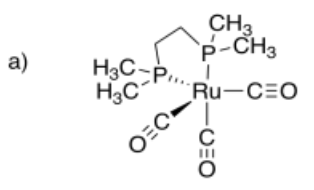# 2.1.6: Naming Transition Metal Complexes

•• Kathryn Haas
• Duke University
$$\newcommand{\vecs}{\overset { \rightharpoonup} {\mathbf{#1}} }$$ $$\newcommand{\vecd}{\overset{-\!-\!\rightharpoonup}{\vphantom{a}\smash {#1}}}$$$$\newcommand{\id}{\mathrm{id}}$$ $$\newcommand{\Span}{\mathrm{span}}$$ $$\newcommand{\kernel}{\mathrm{null}\,}$$ $$\newcommand{\range}{\mathrm{range}\,}$$ $$\newcommand{\RealPart}{\mathrm{Re}}$$ $$\newcommand{\ImaginaryPart}{\mathrm{Im}}$$ $$\newcommand{\Argument}{\mathrm{Arg}}$$ $$\newcommand{\norm}{\| #1 \|}$$ $$\newcommand{\inner}{\langle #1, #2 \rangle}$$ $$\newcommand{\Span}{\mathrm{span}}$$ $$\newcommand{\id}{\mathrm{id}}$$ $$\newcommand{\Span}{\mathrm{span}}$$ $$\newcommand{\kernel}{\mathrm{null}\,}$$ $$\newcommand{\range}{\mathrm{range}\,}$$ $$\newcommand{\RealPart}{\mathrm{Re}}$$ $$\newcommand{\ImaginaryPart}{\mathrm{Im}}$$ $$\newcommand{\Argument}{\mathrm{Arg}}$$ $$\newcommand{\norm}{\| #1 \|}$$ $$\newcommand{\inner}{\langle #1, #2 \rangle}$$ $$\newcommand{\Span}{\mathrm{span}}$$$$\newcommand{\AA}{\unicode[.8,0]{x212B}}$$

## Introduction

It's useful to be able to read the name of a complex or compound and understand what that means. First, let's get straight on some vocabulary:

COMPLEXES (metal directly bonded to ligands)

• Complex Cation: This is a metal complex with an overall positive charge. e.g. $$[CO(NH_3)_6]^{3+}$$
• Complex Anion: This is a metal complex with an overall negative charge. e.g. $$[CoCl_4(NH_3)_2]^-$$
• Neutral Complex: This is a metal complex with zero overall charge. e.g. $$[CoCl_3(NH_3)_3]$$

COMPOUNDS (salt of one or more complexes)

• Coordination Compounds: This is a salt where either the cation and/or the anion are metal complexes. e.g. $$K_4[Fe(CN)_6]$$

## The Rules: Naming Complexes and Coordination Compounds

 1. Naming a coordination compound (salt): Cation first, then anion. Also list cation first in formula. (just like gen chem: NaCl = sodium chloride)2. Naming a complex/complex ion: ligands first, metal second, written as one long word. *in the formula, the complex ion is written in [square brackets] and the metal is written first*if the ligand itself includes one of the prefixes, or is complicated, a second set of prefixes is used.4. Ligands are named in alphabetical order. (see example above) 5. Anionic ligands are given “o” suffix. Neutral Ligands retain usual name. (see example above)6. Oxidation number of transition metals is given by (Roman Numeral). *for negative oxidation numbers, charge is given by (-#) 7. Negatively charged complex ion is given suffix “-ate”.8. Arrangement of ligands must be specified for stereoisomers: Cis / Trans, Δ / Λ , mer / fac.9. Bridging Ligands between metal ions have prefix μn (n=# of atoms bridged, usually dropped if n=2).10. Ambidentate ligands use κ-X## The Rules: Writing Formulas of Coordination Complexes

The formula of a coordination complex is written in a different order than its name. The chemical symbol of the metal center is written first. The ligands are written next, with anion ligands coming before neutral ligands. If there is more than one anion or neutral ligand, they are written in alphabetical order according to the first letter in their chemical formula.In a coordination compound's name, when one of the ions is just an element, the number of atoms is not indicated with a prefix. Since it still has to be written in the formula, it is determined by balancing the overall charge of the compound. (For example, tetrafluorochromium(VI) chloride becomes [CrF4]Cl2.

##### Exercise $$\PageIndex{1}$$

Provide names for the following complexes:

a) K[Cr(ox)2(OH2)2] b) [Co(NH3)5Br](NO3)2 c) [Cr(en)2Cl2]PF6 d) [Co(bpy)2(OH)Cl]ClO4

e) [TiCl3(OH2)3] f) K3[Fe(CN)6] g) Na[Au(bpy)(CN)2]

potassium diaquabis(oxalato)chromate(III)

pentaamminebromocobalt(III) nitrate

dichlorobis(ethylenediamine)chromium(III) hexafluorophosphate

bis(bipyridine)chlorohydroxocobalt(III) perchlorate

triaquatrichlorotitanium(III)

potassium hexacyanoferrate(III)

sodium bipyridinedicyanoaurate(I)

Bipyridine is sometimes called "bipyridyl".

##### Exercise $$\PageIndex{2}$$

Draw the following structures:

a) dicarbonylbis(1,2-dimethylphosphino)ethaneruthenium (0)

b) potassium bipyridyltetracyanoferrate(II)

c) pentaamminechlorochromium(III) perchlorate

d) tetraacetonitrilecopper(I) tetrafluoroborate

e) sodium ethylenediaminebis(oxalato)cobalt(III)

f) chlorotris(triphenylphosphine)rhodium(I)